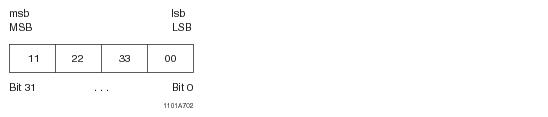# 15.00 - Bit and Byte Numbering Model - Teradata Database

## Teradata Database SQL Functions, Operators, Expressions, and Predicates

Product
Release Number
15.00
Content Type
Programming Reference
Publication ID
B035-1145-015K
Language
English (United States)

## Bit and Byte Numbering Model

The following diagrams show the logical bit and byte numbering model employed by the byte/bit manipulation functions described in this chapter.

The model is big endian or little endian independent. Note that the numbering system used for numeric data types is consistent with that used for byte strings. This simplifies the development of appropriate bit masks.

Users of the byte/bit manipulation functions should mentally visualize the numeric and byte data types as shown below when contemplating what masks (bit_mask_arg) need to be applied to the target data (target_arg).

### BYTEINT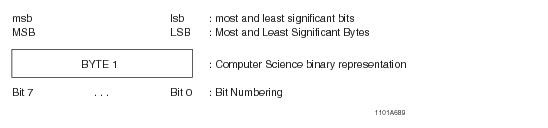#### Example

A BYTEINT value of 40 with a binary representation of 00101000: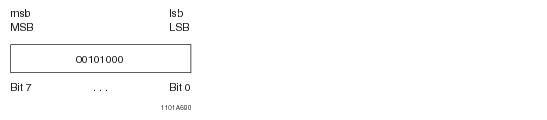### SMALLINT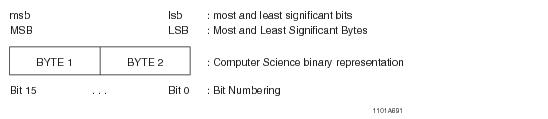#### Example

A SMALLINT value of 10,280 with a binary representation of 0010100000101000: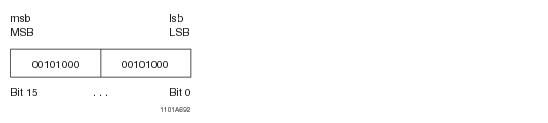### INTEGER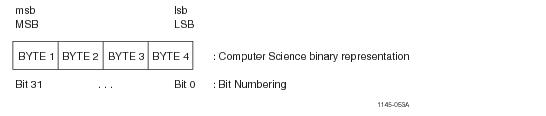#### Example

An INTEGER value of 673,720,360 with a binary representation of
00101000 00101000 00101000 00101000: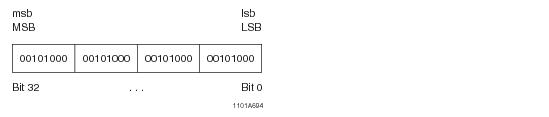### BIGINT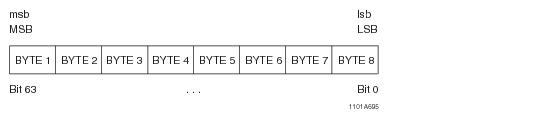#### Example

A BIGINT value of 2,893,606,913,523,066,920 with a binary representation of
00101000 00101000 00101000 00101000 00101000 00101000 00101000 00101000: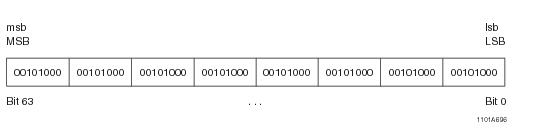### BYTE and VARBYTE

#### Example

A VARBYTE(8) with 8 bytes: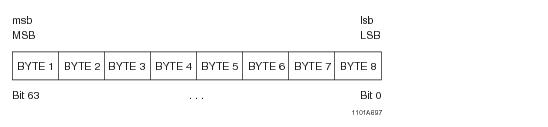#### Example

A VARBYTE(8) with 3 bytes: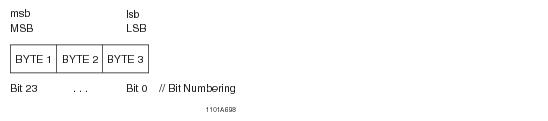#### Example

Example of BYTE(4):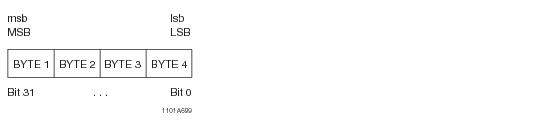With respect to byte-bit system functions, hexadecimal byte literals are interpreted as follows:

A 2-byte hexadecimal byte literal: '00FF'XB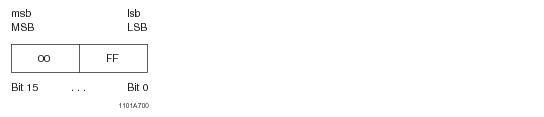A 4-byte hexadecimal byte literal: '01020304'XB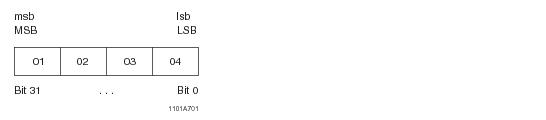Note that hexadecimal byte literals are represented by an even number of hexadecimal digits. Hexadecimal literals are extended on the right with zeros when required. For example:

A 3-byte hexadecimal byte literal, '112233'XB, becomes a 4-byte hexadecimal byte literal: '11223300'XB